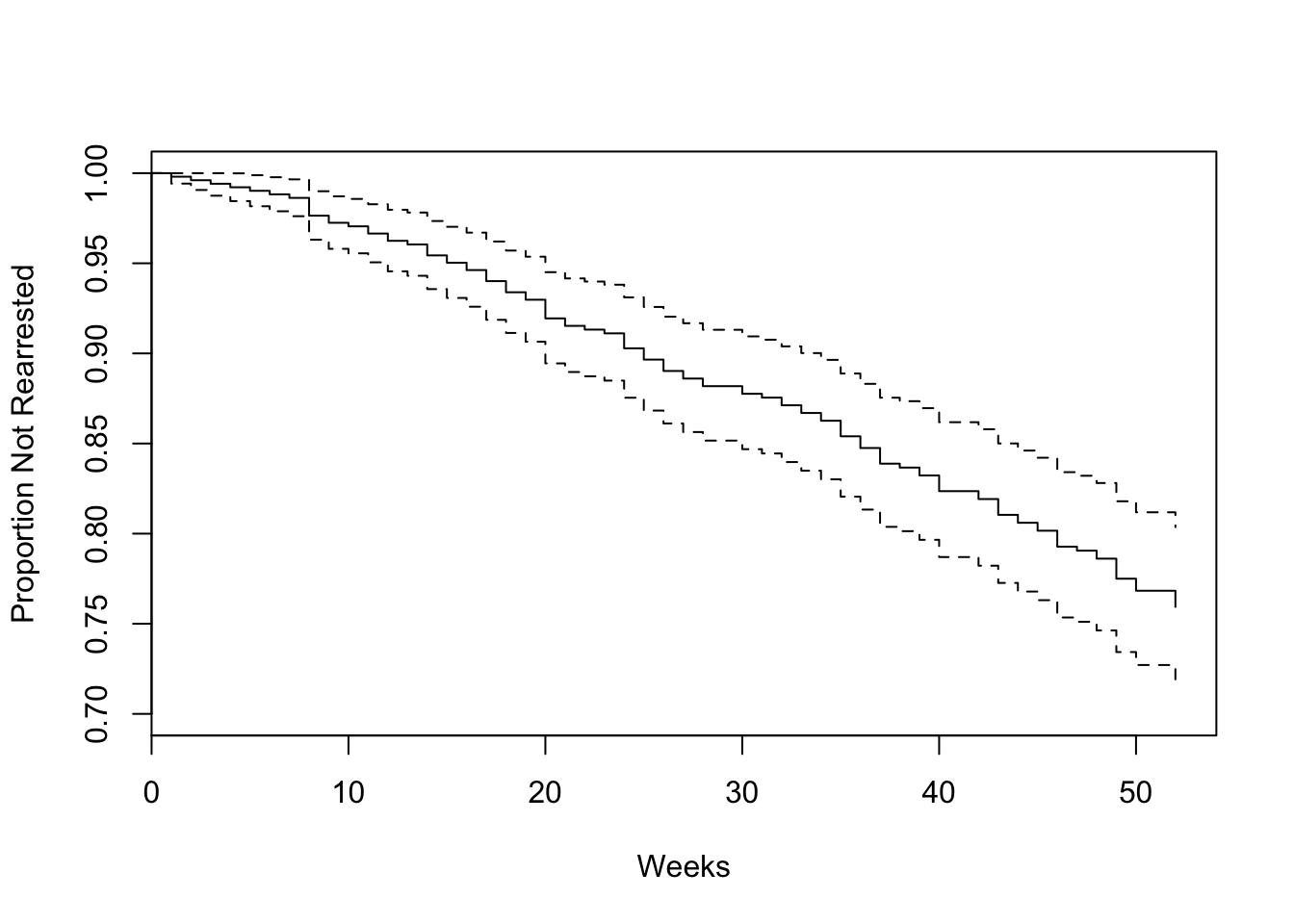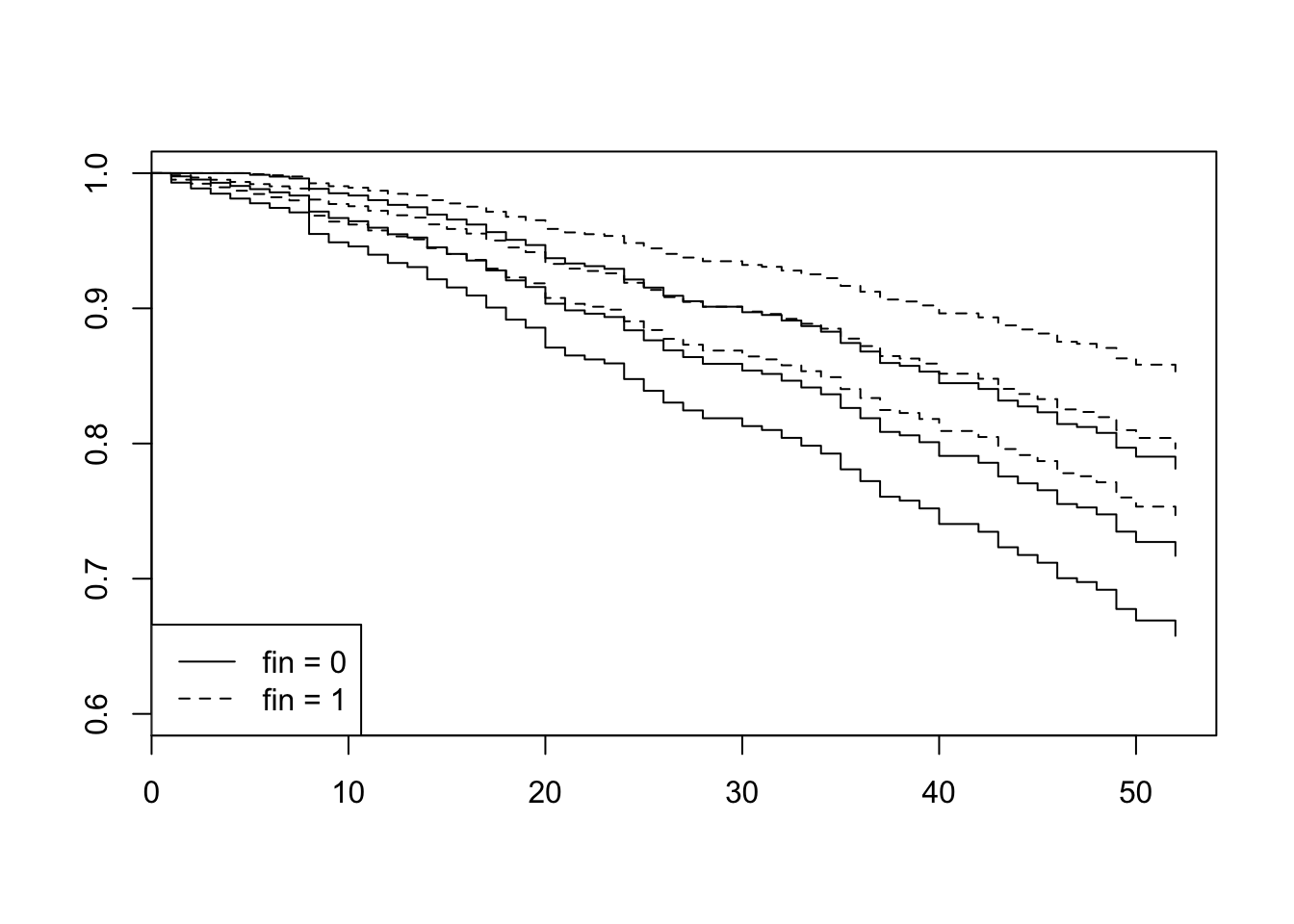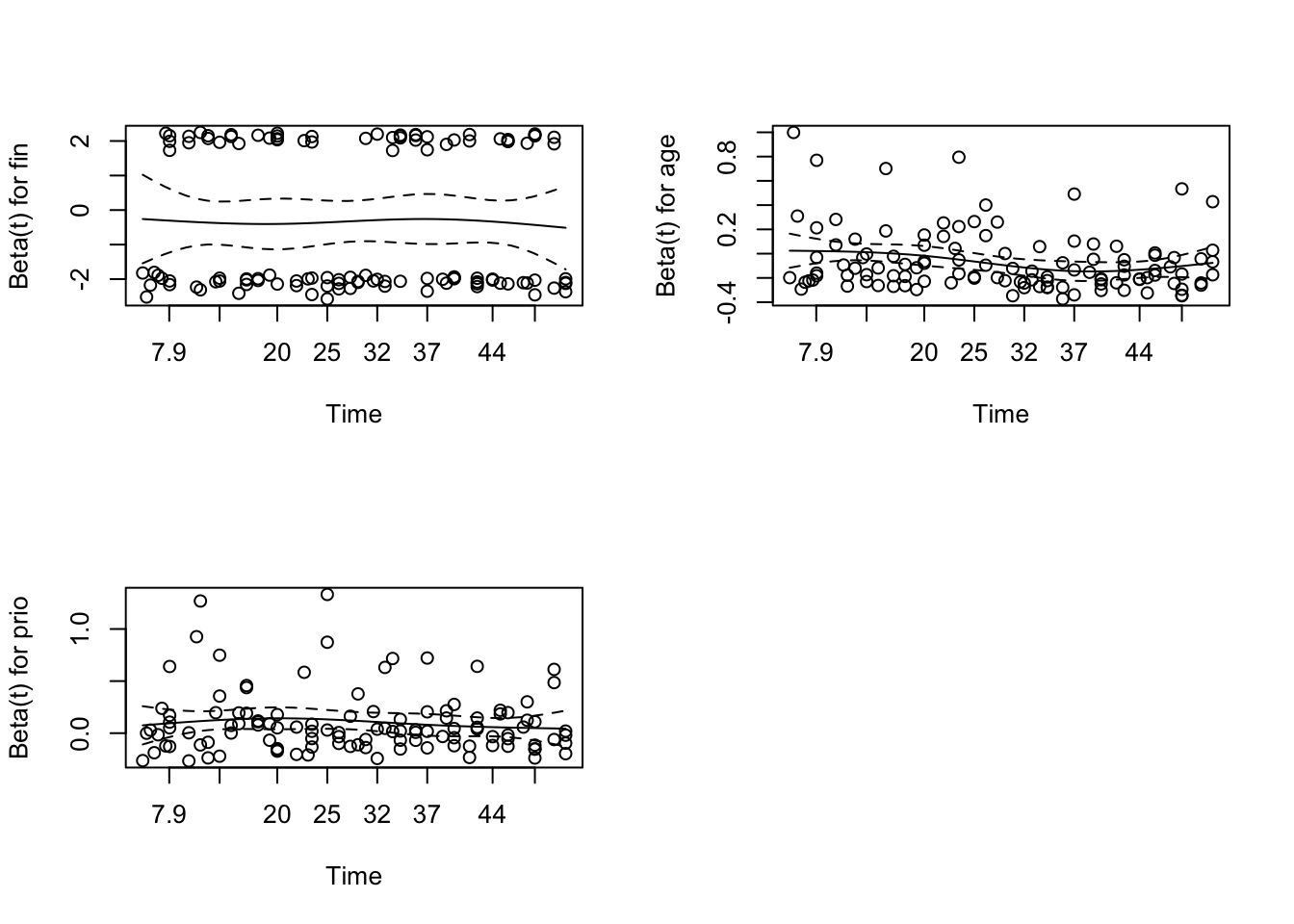# 笔记 21 生存分析

## 21.1 Concepts

• examines and models the time it takes for events to occur
• the distribution of survival times
• Popular: Cox proportional-hazards regression model

## 21.2 Notation

• T as a random variable with cumulative distribution function $$P (t) = Pr(T ≤ t)$$ and probability density function $$p(t) = \frac{dP (t)}{dt}$$
• survival function S(t) is the complement of the distribution function, $$S(t) = Pr(T > t) = 1 − P (t)$$
• hazard function $$log h(t) = ν + ρt$$

## 21.3 Cox proportional-hazards regression model

• $$log h_i(t)=α+_1x_{i1} +β_2x_{ik} +···+β_kx_{ik}$$
• Cox model
• $$log h_i(t)=α(t)+β_1x_{i1} +β_2x_{ik} +···+β_kx_{ik}$$
• the Cox model is a proportional-hazards model
• $$\frac{h_i(t)}{h_{i'}(t)} = \frac{e^{\eta_i}}{e^{\eta'}}$$

## 21.4 Case: Recidivism

• Target: recidivism of 432 male prisoners, who were observed for a year after being released from prison
• arrest means the male prisoners who rearrested
• 52 weeks
• factors: financial aid after release from prison, affected，release ages，race，work experience，marriage，parole，prior convictions, education
library(survival)
library(car)
# perform survival analysis
Rossi[1:5, 1:10]
##   week arrest fin age race wexp mar paro prio educ
## 1   20      1   0  27    1    0   0    1    3    3
## 2   17      1   0  18    1    0   0    1    8    4
## 3   25      1   0  19    0    1   0    1   13    3
## 4   52      0   1  23    1    1   1    1    1    5
## 5   52      0   0  19    0    1   0    1    3    3
mod.allison <- coxph(Surv(week, arrest) ~ fin + age + race + wexp + mar + paro + prio, data=Rossi)
summary(mod.allison)
## Call:
## coxph(formula = Surv(week, arrest) ~ fin + age + race + wexp +
##     mar + paro + prio, data = Rossi)
##
##   n= 432, number of events= 114
##
##         coef exp(coef) se(coef)     z Pr(>|z|)
## fin  -0.3794    0.6843   0.1914 -1.98   0.0474 *
## age  -0.0574    0.9442   0.0220 -2.61   0.0090 **
## race  0.3139    1.3688   0.3080  1.02   0.3081
## wexp -0.1498    0.8609   0.2122 -0.71   0.4803
## mar  -0.4337    0.6481   0.3819 -1.14   0.2561
## paro -0.0849    0.9186   0.1958 -0.43   0.6646
## prio  0.0915    1.0958   0.0286  3.19   0.0014 **
## ---
## Signif. codes:  0 '***' 0.001 '**' 0.01 '*' 0.05 '.' 0.1 ' ' 1
##
##      exp(coef) exp(-coef) lower .95 upper .95
## fin      0.684      1.461     0.470     0.996
## age      0.944      1.059     0.904     0.986
## race     1.369      0.731     0.748     2.503
## wexp     0.861      1.162     0.568     1.305
## mar      0.648      1.543     0.307     1.370
## paro     0.919      1.089     0.626     1.348
## prio     1.096      0.913     1.036     1.159
##
## Concordance= 0.64  (se = 0.027 )
## Rsquare= 0.074   (max possible= 0.956 )
## Likelihood ratio test= 33.3  on 7 df,   p=2.36e-05
## Wald test            = 32.1  on 7 df,   p=3.87e-05
## Score (logrank) test = 33.5  on 7 df,   p=2.11e-05
# plot time vs survival prob
plot(survfit(mod.allison), ylim=c(.7, 1), xlab='Weeks', ylab='Proportion Not Rearrested')### 21.4.1 result

• The covariates age and prio (prior convictions) have highly statistically significant coefficients, while the coefficient for fin (financial aid) is marginally significant
• holding the other covariates constant, an additional year of age reduces the weekly hazard of rearrest by a factor of $$e^b = 0.944$$ on average – that is, by 5.6
• likelihood-ratio, Wald, and score chi-square statistics: null hypothesis all of the β’s are zero.

## 21.5 further

• assess the impact of financial aid on rearrest
• new data frame with two rows, one for each value of fin; the other covariates are fixed to their average values
attach(Rossi)
Rossi.fin <- data.frame(fin=c(0,1), age=rep(mean(age),2), race=rep(mean(race),2), wexp=rep(mean(wexp),2), mar=rep(mean(mar),2), paro=rep(mean(paro),2), prio=rep(mean(prio),2))
detach()
plot(survfit(mod.allison, newdata=Rossi.fin), conf.int=T, lty=c(1,2), ylim=c(.6, 1))
legend("bottomleft", legend=c('fin = 0', 'fin = 1'), lty=c(1,2))• the higher estimated ‘survival’ of those receiving financial aid, but the two confidence envelopes overlap substantially, even after 52 weeks

## 21.6 Time-Dependent Covariates

• treat the employed variable as a tim-dependent covariates with 52 weeks’ record
sum(!is.na(Rossi[,11:62])) # record count
##  19809
Rossi2 <- matrix(0, 19809, 14) # to hold new data set
colnames(Rossi2) <- c('start', 'stop', 'arresttime', names(Rossi)[1:10], 'employed')

row<-0
for (i in 1:nrow(Rossi)) {
for (j in 11:62) {
if (is.na(Rossi[i, j])) next
else {
row <- row + 1 # increment row counter
start <- j - 11 # start time (previous week)
stop <- start + 1 # stop time (current week)
arresttime <- if (stop == Rossi[i, 1] && Rossi[i, 2] ==1) 1 else 0
Rossi2[row,] <- c(start, stop, arresttime, unlist(Rossi[i, c(1:10, j)]))
}
}
}
Rossi2 <- as.data.frame(Rossi2)
remove(i, j, row, start, stop, arresttime)
modallison2 <- coxph(Surv(start, stop, arresttime) ~ fin + age + race + wexp + mar + paro + prio + employed, data=Rossi2)
summary(modallison2)
## Call:
## coxph(formula = Surv(start, stop, arresttime) ~ fin + age + race +
##     wexp + mar + paro + prio + employed, data = Rossi2)
##
##   n= 19809, number of events= 114
##
##             coef exp(coef) se(coef)     z Pr(>|z|)
## fin      -0.3567    0.7000   0.1911 -1.87   0.0620 .
## age      -0.0463    0.9547   0.0217 -2.13   0.0330 *
## race      0.3387    1.4031   0.3096  1.09   0.2740
## wexp     -0.0256    0.9748   0.2114 -0.12   0.9038
## mar      -0.2937    0.7455   0.3830 -0.77   0.4431
## paro     -0.0642    0.9378   0.1947 -0.33   0.7416
## prio      0.0851    1.0889   0.0290  2.94   0.0033 **
## employed -1.3283    0.2649   0.2507 -5.30  1.2e-07 ***
## ---
## Signif. codes:  0 '***' 0.001 '**' 0.01 '*' 0.05 '.' 0.1 ' ' 1
##
##          exp(coef) exp(-coef) lower .95 upper .95
## fin          0.700      1.429     0.481     1.018
## age          0.955      1.047     0.915     0.996
## race         1.403      0.713     0.765     2.574
## wexp         0.975      1.026     0.644     1.475
## mar          0.745      1.341     0.352     1.579
## paro         0.938      1.066     0.640     1.374
## prio         1.089      0.918     1.029     1.152
## employed     0.265      3.775     0.162     0.433
##
## Concordance= 0.708  (se = 0.027 )
## Rsquare= 0.003   (max possible= 0.066 )
## Likelihood ratio test= 68.7  on 8 df,   p=9.11e-12
## Wald test            = 56.1  on 8 df,   p=2.63e-09
## Score (logrank) test = 64.5  on 8 df,   p=6.1e-11

## 21.7 Model Diagnostics

• Checking Proportional Hazards
modallison3 <- coxph(Surv(week, arrest) ~ fin + age + prio, data=Rossi)
modallison3
## Call:
## coxph(formula = Surv(week, arrest) ~ fin + age + prio, data = Rossi)
##
##         coef exp(coef) se(coef)     z       p
## fin  -0.3470    0.7068   0.1902 -1.82 0.06820
## age  -0.0671    0.9351   0.0209 -3.22 0.00129
## prio  0.0969    1.1017   0.0273  3.56 0.00038
##
## Likelihood ratio test=29.1  on 3 df, p=2.19e-06
## n= 432, number of events= 114
cox.zph(modallison3)
##             rho   chisq      p
## fin    -0.00657 0.00507 0.9433
## age    -0.20976 6.54147 0.0105
## prio   -0.08004 0.77288 0.3793
## GLOBAL       NA 7.13046 0.0679
par(mfrow=c(2,2))
plot(cox.zph(modallison3))• there appears to be a trend in the plot for age, with the age effect declining with time
modallison4 <- coxph(Surv(start,stop,arresttime)~fin+age+age:stop:stop+prio, data = Rossi2)
modallison4
## Call:
## coxph(formula = Surv(start, stop, arresttime) ~ fin + age + age:stop:stop +
##     prio, data = Rossi2)
##
##              coef exp(coef) se(coef)     z       p
## fin      -0.34856   0.70570  0.19023 -1.83 0.06690
## age       0.03228   1.03280  0.03943  0.82 0.41301
## prio      0.09818   1.10316  0.02726  3.60 0.00032
## age:stop -0.00383   0.99617  0.00147 -2.61 0.00899
##
## Likelihood ratio test=36  on 4 df, p=2.85e-07
## n= 19809, number of events= 114
• the coefficient for the interaction is negative and highly statistically significant: The effect of age declines with time

• use residual to find influential observations